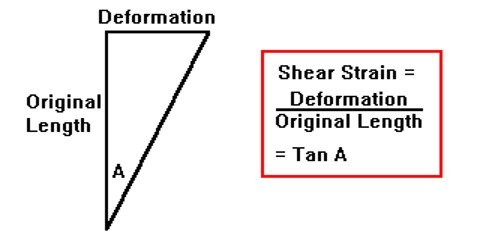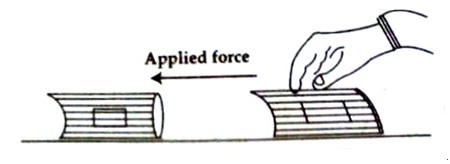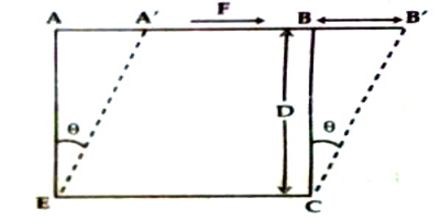# What is Shearing Strain?

Shearing strain: If due to the action of the applied force the shape of an object changes but not the volume or if the object gets twisted then that type of strain is called shearing strain. It is the ratio of the displacement of a layer to its distance from the fixed layer. As a result of this, the stress developed within the body is called shearing stress.

Example: If a thick book, fixed at its lower face on the table be pushed tangentially on the upper face of the book, then it will be seen that the shape of the book has been changed (Figure 1). Due to the action of the applied force each page of the upper part has shifted slightly relative to the lower face. It is Shearing strain. In the figure, a rectangle drawn on the side face has been changed to a parallelogram.Shearing strain is measured by the angular deformation caused due to change of shape of shape.

Explanation: Let ABCE be a cube (Figure 2). By keeping the side CE fixed a tangential force ‘F’ is applied on side AB. Due to the action of the applied force let points A and B be shifted to A′ and B′ respectively. Now, the original shape of cube ABCE has become a rhombus A′B′CE. So it is seen that the shape of the object has been changed. This is called shearing strain.This shearing strain as measured by the angular displacement. Let the angular displacement = θ, which is very small,

Shearing strain, θ = d/D     (as we know, θ = tan θ = d/D)

Here, AA′ = BB′ = d and BC = AE= D

So, shearing strain = [Relative displacement / Intervening distance]